# Rayleigh-Ritz method

The Rayleigh-Ritz method is used for the computation of approximate solutions of operator eigenvalue equations and partial differential equations. The method is based on a linear expansion of the solution and determines the expansion coefficients by a variational procedure, which is why the method is also known as linear variation method.

The method is named for the Swiss mathematical physicist Walter Ritz and the English physicist Lord Rayleigh (John William Strutt). Among numerical mathematicians it is common to append the name of the Russian mathematican Boris Galerkin and to refer to it as the Rayleigh-Ritz-Galerkin method.

## The method

The method is widely used in quantum mechanics where the central equation—the time-independent Schrödinger equation—has the form of an eigenvalue equation of an operator commonly denoted by H, the Hamilton (or energy) operator. The operator H is Hermitian and contains second derivatives. The Rayleigh-Ritz method applied to the eigenvalue problem of H will be discussed in this section. The results can be transferred fairly easily to similar non-quantum mechanical eigenvalue problems and certain partial differential equations.

The aim is to formulate an approximate method for solving the eigenvalue equation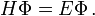$H\Phi = E \Phi\,.$

It will be shown that this equation can be replaced by the problem of finding a stationary point of the expectation value of H with respect to Φ: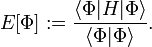$E[\Phi] := \frac{\langle \Phi | H | \Phi \rangle}{ \langle \Phi |\Phi \rangle}.$

Note that this is a map of the function Φ onto the number E[Φ], i.e., we have here a mathematical construct known as a functional. The bra-ket notation ⟨..|..|..⟩ implies the integration over a configuration space, which in electronic structure theory usually is ℝ3N with N being the number of electrons of the system described by the Hamiltonian H. We assume boundary conditions on Φ and integration limits such that E[Φ] is finite and the Hamiltonian H is Hermitian. In that case E[Φ] is a real number. The boundary condition in electronic structure theory is the vanishing of Φ at infinite interparticle distances and the 3N integrations, implied by the bracket, are from minus infinity to plus infinity. Bounded configuration spaces with periodic boundary conditions also occur in quantum mechanics and variational Rayleigh-Ritz theory, outlined below, applies to these cases as well.

### The variational principle

Most non-relativistic Hamiltionians in quantum mechanics have a lower bound, that is there is a finite real number B such that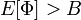$E[\Phi] > B \,$

for all admissible Φ. The function Φ is admissible when the functional E[Φ] is well-defined (for instance Φ must be twice differentiable) and Φ has the correct boundary conditions. We assume from now on that H has a lower bound; if this is not the case the Rayleigh-Ritz method is not applicable.

The highest lower bound is the lowest eigenvalue E0 of H. To show this we assume that the eigenvalues and eigenvectors of H are known and moreover that the eigenvectors are orthonormal and complete (form an orthonormal basis of Hilbert space),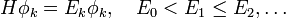$H\phi_k = E_k \phi_k, \quad E_0 < E_1 \le E_2, \dots$

where for convenience sake we assume the ground state to be non-degenerate and the eigenvectors to be countable. Because H is bounded from below, E0 := E0] > B is finite. Completeness and orthonormality gives the resolution of the identity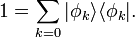$1 = \sum_{k=0} | \phi_k\rangle \langle \phi_k | .$

Inserting this resolution into the functional at appropriate places, and using that the Hamilton matrix on basis of eigenvectors is diagonal with Ek on the diagonal, yields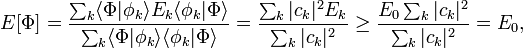$E[\Phi] = \frac{\sum_k \langle\Phi|\phi_k\rangle E_k \langle \phi_k |\Phi\rangle} {\sum_k \langle\Phi|\phi_k\rangle \langle \phi_k |\Phi\rangle} = \frac{\sum_k |c_k|^2 E_k } {\sum_k |c_k|^2} \ge \frac{E_0\sum_k |c_k|^2 } {\sum_k |c_k|^2} = E_0,$

where ck ≡ ⟨ φk | Φ ⟩.

This result states that any expectation value is larger than or equal to the lowest eigenvalue E0. The lowest expectation value E[Φ] of H is obtained if we take Φ = φ0, because it is then equal to E0. If, conversely, we find a Φ such that E[Φ] = E0, it can be concluded that Φ is the exact eigenfunction of lowest energy, because supposing the opposite gives a contradiction: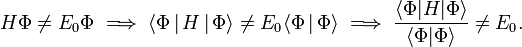$H\Phi \ne E_0 \Phi \;\Longrightarrow\; \langle \Phi\,|\,H\,|\,\Phi\rangle \ne E_0 \langle \Phi\,|\,\Phi\rangle \;\Longrightarrow\; \frac{\langle \Phi|H|\Phi\rangle} {\langle \Phi|\Phi\rangle}\ne E_0.$

In total: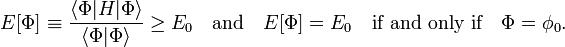$E[\Phi]\equiv\frac{\langle \Phi|H|\Phi\rangle} {\langle \Phi|\Phi\rangle}\ge E_0 \quad\hbox{and}\quad E[\Phi] = E_0 \quad\hbox{if and only if}\quad \Phi=\phi_0.$

This result is referred to as the variational principle.

### Matrix eigenvalue problem

It will be convenient to rewrite the energy functional in the Lagrange form of undetermined multipliers. That is, redefine the functional as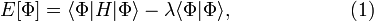$E[\Phi] = \langle \Phi | H | \Phi \rangle - \lambda \langle \Phi | \Phi \rangle,\qquad\qquad\qquad(1)$

where λ is an undetermined multiplier. It can be shown that this functional has the same stationary values for the same functions Φ as the original functional. Minimizing the Lagrange form is completely equivalent to minimizing the Rayleigh quotient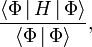$\frac{\langle \Phi \,|\, H \,|\, \Phi \rangle}{\langle \Phi \,|\, \Phi \rangle},$

but the former procedure requires slightly less algebra.

Take now an arbitrary, non-complete, possibly non-orthogonal, expansion set χk, and approximate Φ by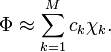$\Phi \approx \sum_{k=1}^M c_k \chi_k.$

One gets the approximation for the functional in Eq. (1),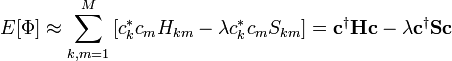$E[\Phi] \approx \sum_{k, m=1}^M \left[ c_k^* c_m H_{km} - \lambda c_k^* c_m S_{km} \right] = \mathbf{c}^\dagger \mathbf{H} \mathbf{c} - \lambda \mathbf{c}^\dagger \mathbf{S} \mathbf{c}$

with the M × M matrices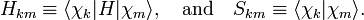$H_{km} \equiv \langle \chi_k | H | \chi_m \rangle, \quad\hbox{and}\quad S_{km} \equiv \langle \chi_k | \chi_m \rangle.$

Stationary points—such as minima—of the functional are obtained from equations in which derivatives are put equal to zero,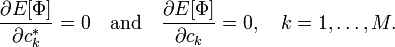$\frac{\partial E[\Phi]}{\partial c_k^*} = 0 \quad \hbox{and}\quad \frac{\partial E[\Phi]}{\partial c_k} = 0, \quad k=1,\ldots, M.$

The ensuing equations can be formulated in matrix notation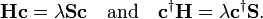$\mathbf{H} \mathbf{c} = \lambda \mathbf{S} \mathbf{c} \quad \hbox{and}\quad \mathbf{c}^\dagger \mathbf{H} = \lambda \mathbf{c}^\dagger \mathbf{S}.$

These two equations are the same (follow from each other by Hermitian conjugation) because the matrices H and S are Hermitian and λ is real. Hence, a stationary point of the approximate functional is obtained by solution of the generalized matrix eigenvalue equation,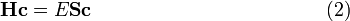$\mathbf{H} \mathbf{c} = E \mathbf{S} \mathbf{c} \qquad\qquad\qquad\qquad\qquad\qquad (2)$

where we changed the notation from λ to E.

Make now two assumptions: the set χk is complete and the set is orthonormal. (The second assumption is non-essential, but simplifies the notation). In an orthonormal basis the following simple expression holds for the expansion coefficients,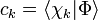$c_k = \langle \chi_k | \Phi \rangle$

Note, parenthetically, that in general completeness of the expansion requires infinite M. Introduce the resolution of identity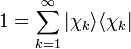$1 =\sum_{k=1}^\infty |\chi_k\rangle \langle \chi_k|$

into$\sum_k \langle \chi_m|H|\chi_k\rangle \langle \chi_k|\Phi \rangle = E \sum_k \delta_{mk} \langle \chi_k|\Phi \rangle \quad \Longrightarrow \quad \langle \chi_m|H|\Phi \rangle = \langle \chi_m|\Phi \rangle, \quad m=1,2,\ldots, \infty.$

Multiply the equation after the arrow on the left by |χm ⟩, sum over m, and use the resolution of the identity again, then we find the result that we set out to prove: In a complete basis the generalized eigenvalue problem (2) is equivalent to$H\Phi = E \Phi\,.$

and the determination of stationary points of the functional E[Φ] by solution of the (infinite-dimensional) matrix eigenvalue problem is equivalent to solving the operator eigenvalue equation. Ritz's important insight was that expanding a trial function in a finite-dimensional non-complete basis χk and minimizing the expectation value of H, gives an approximation to the lowest eigenvector of H.

Summary of the procedure

The Rayleigh-Ritz variational method starts by choosing an expansion basis χk of dimension M. This expansion is inserted into the energy functional [in its Lagrange form, Eq. (1)] and variation of the coefficients gives the generalized matrix eigenvalue problem (2). The solution of this problem yields stationary points (usually minima). The lowest eigenvector approximates the lowest exact solution of the operator eigenvalue equation to be solved (the time-independent Schrödinger equation). The success of the method relies to a large extent on the appropriateness of the expansion basis. When the expansion basis approaches completeness, the solution vector approaches the exact solution of the operator eigenvalue equation.

## History

In the older quantum mechanics literature the method is known as the Ritz method, called after the mathematical physicist Walter Ritz, who first devised it. In prewar quantum mechanics texts it was customary to follow the highly influential book by Courant and Hilbert, who were contemporaries of Ritz and write of the Ritz procedure (Ritzsches Verfahren).

In the numerical analysis and mechanical engineering literature one usually prefixes the name of Lord Rayleigh to the method, and lately this has become common in quantum mechanics, too. Leissa, knowing the method from applications in mechanical engineering, recently became intrigued by the name and after reading the original sources, he discovered that the methods of the two workers differ considerably, although Rayleigh himself believed that the methods were very similar and that his own method predated the one of Ritz by several decades. However, according to Leissa's convincing conclusion, Rayleigh was mistaken and the method now known as Rayleigh-Ritz method is solely due to Ritz. Leissa states:

Therefore, the present writer concludes that Rayleigh’s name should not be attached to the Ritz method; that is, the Rayleigh–Ritz method is an improper designation.